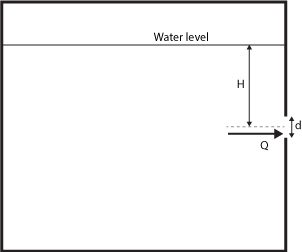# Orifice Flow Calculator

Created by Rahul Dhari
Reviewed by Steven Wooding
Last updated: Feb 06, 2022

The orifice flow calculator estimates the flow rate of liquid discharged from a tank through an orifice. In plain and simple terms, the orifice is a hole or a cutout, using which, one can control or restrict the flow rate.

An orifice meter measures and controls the mass flow rate through an orifice for liquids in a tank and is extensively used in fluid mechanics allied applications such as hydraulic equipment, compressors, water tanks, sewage, weirs, and spillways. Orifices are also used to create pressure differentials in a flow.

Read on to understand how does this calculator assists you in determining the mass flow through an orifice.

## What is an orifice and orifice flow rate?

An orifice is a plate with a hole or cutout attached to the outlet or inserted inside a pipe, such that the flow passes through the orifice. The orifice, usually circular, is of known diameter and area, to keep the flow regulated. As the fluid flows through an orifice, the pressure drops across the pipe. This phenomenon is also used to measure the fluid characteristics.

Mathematically, the orifice flow equation gives the mass flow rate, or discharge Q through an orifice, given the area of the orifice A, and can be written as:

Q = Cd * A * √(2 * g * H)

where, Cd is coefficient of discharge, g is acceleration due to gravity in m/s2, and H being the mean center line (distance between the water level and the center of the orifice).

The coefficient of discharge, Cd is a function of various parameters such as the diameter of orifice d, acceleration due to gravity, hydraulic depth, and the kinematic viscosity of the fluid ν. Mathematically, it can be expressed as:

Cd ∝ (d * √(g * H) / ν)

## How to calculate orifice flow rate?

To estimate the orifice flow rate, using the orifice equation discussed above:

1. Insert the diameter of the orifice, d.
2. The calculator will now calculate the area of orifice, A.
3. Enter the coefficient of discharge, Cd.
4. Enter the value of the mean center line, H.
5. The acceleration due to gravity, g is filled in as 9.81 m/s2 for your convenience. It can be modified using the advanced mode of the calculator.
6. The orifice flow calculator will now return the value of discharge or orifice flow rate, Q.

## Example: Using the orifice flow calculator

Determine the flow rate through the orifice having a diameter of 50 mm and mean center line as 200 mm. Take coefficient of discharge, Cd as 0.8.

To estimate the orifice flow rate:

1. Insert the diameter of the orifice, d = 50 mm.
2. The calculator will now estimate the area of orifice, A.
3. Enter the coefficient of discharge, Cd = 0.8.
4. Enter the value of the mean center line, H = 200 mm.
5. The acceleration due to gravity, g is filled in as 9.81 m/s2.
6. Using the orifice equation:
Q = Cd * A * √(2 * g * H)
Q = 0.8 * 0.0019635 * √(2 * 9.81 * 0.2) = 0.0031 m3/s

## FAQ

### What is an orifice?

An orifice consists of a flat plate with a cutout that is fixed inside a pipe or at an outlet to create a pressure differential in the fluid flow.

### What is orifice flow rate?

The orifice flow rate is defined as the volume of fluid flow through an orifice per unit time. Mathematically, it can be written using the orifice flow equation:

Q = Cd × A × √(2 × g × H)

### How to calculate mass flow rate through an orifice?

To calculate the flow rate of fluid passing through an orifice:

1. Multiply the gravitational constant g with the mean centerline H.
2. Multiply the produce with 2.
3. Find the square root of the product.
4. Multiply the resultant with the area of orifice A and coefficient of discharge Cd.

### What are the factors influencing coefficient of discharge?

The coefficient of discharge is a function of variables such as the diameter of orifice d, acceleration due to gravity g, hydraulic depth H, and kinematic viscosity of the fluid, ν. Mathematically, we can write:

Cd ∝ (d × √(g × H) / ν)
Rahul DhariDiameter of orifice (d)
in
Area of orifice (A)
in²
Coefficient of discharge (Cd)
in
Discharge (Q)
ft³/s
People also viewed…

### Cyclotron Frequency

Use the Cyclotrone Frequency Calculator to explore the world of the first particle's accelerators.

### Eggs

Quantum physicist's take on boiling a perfect egg.

### Electron speed

The electron speed calculator helps you estimate both the classical and relativistic speeds of an electron.